# How to change error result calculation when using Vlookup formula to 0 or empty cell in Microsoft Excel

Sometimes, we get error or zero as result while applying the Vlookup formula. We get #N/A error when all the lookup values are not available in the data, and we get zero when the lookup value is available but the pick cell is blank.

In this article, we are going to learn how we can change result while applying the Vlookup formula in Microsoft Excel.

To solve this problem, we will use IF, ISERROR and Vlookup functions.

ISERROR function helps us to check the error and will return result as true or false.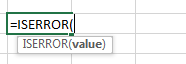IF function will give the condition on the basis of ISERROR function.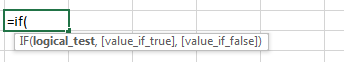Let’s take an example to understand:-

We have 2 data sets of employees. In the first data, we have details, like date of joining, employee name, and designation, and in 2nd data, we have only employee codes and we want to pick the date of joining through Vlookup function.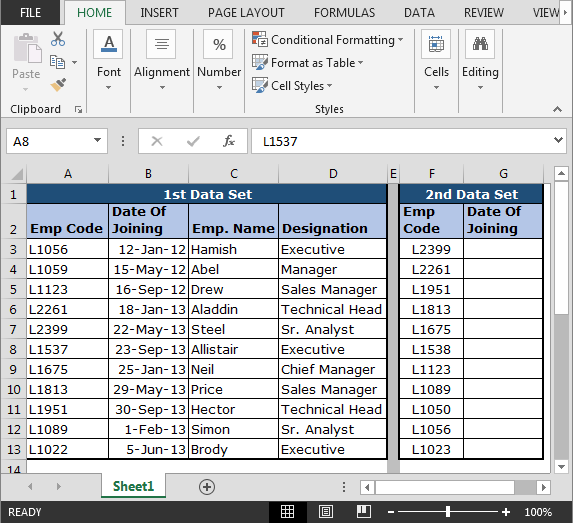Let’s start by using the Vlookup function in data:-

• Enter the formula in cell G2
• ```=VLOOKUP(\$F3,\$A\$3:\$D\$13,2,FALSE)
```
• Press Enter.
• Copy the formula in the rest of cells.Now you can see in the above image that few cells are having the result, but few cells are having #N/A error and first cell is showing 0.

To avoid error, use the function as per the below procedure:-

• Enter the formula in cell H2
• ```=IF(ISERROR(VLOOKUP(\$F3,\$A\$3:\$D\$13,2,FALSE)),"",VLOOKUP(\$F3,\$A\$3:\$D\$13,2,FALSE))
```
• Press Enter
• Copy the formula in the rest of cells.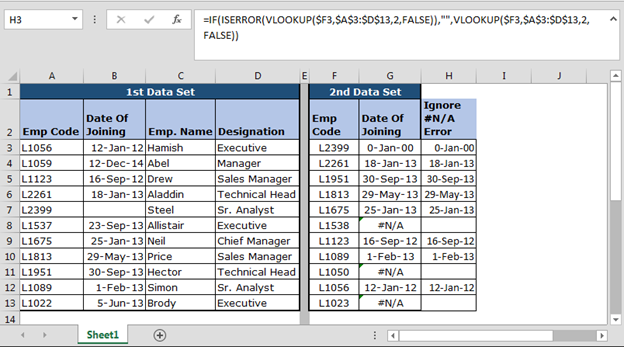Now you can see that instead of showing errors, cells are appearing blank.

To avoid zero, use the function as per the below procedure:-

• Enter the formula in cell H2
• ```=IF(VLOOKUP(\$F3,\$A\$3:\$D\$13,2,FALSE)=0,"",VLOOKUP(\$F3,\$A\$3:\$D\$13,2,FALSE)
```
• Press Enter
• Copy the formula in the rest of cells.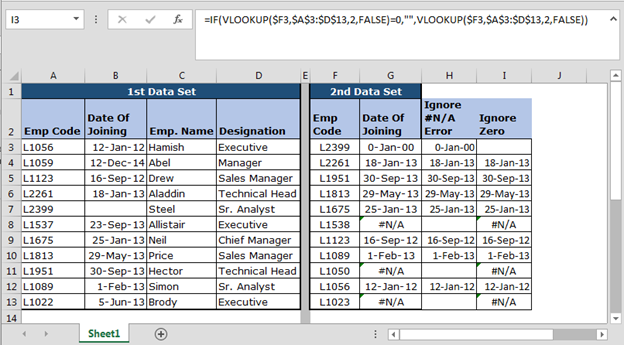To avoid zero and error together, apply below function:-

• Enter the formula in cell H2
• ```=IF(ISERROR(VLOOKUP(\$F3,\$A\$3:\$D\$13,2,FALSE)),"-",IF(VLOOKUP(\$F3,\$A\$3:\$D\$13,2,FALSE)=0,"-",VLOOKUP(\$F3,\$A\$3:\$D\$13,2,FALSE)))
```
• Press Enter
• Copy the formula in the rest of cells.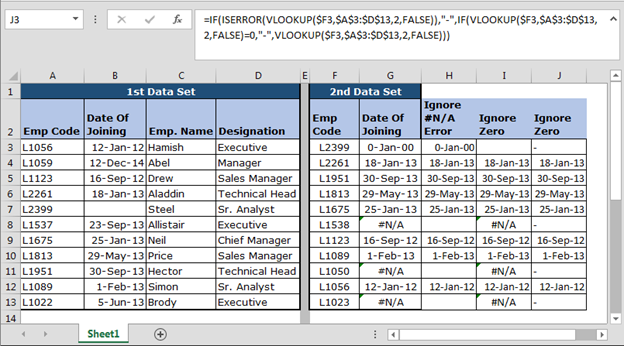In this way, we can use functions to solve our queries about Microsoft Excel.

Note: - In later version of Microsoft Excel 2003, we have IFERROR function in order to get the same result. This formula is compatible with all Microsoft Excel’s version.

1.